# Interactive Analytics of (Large) Financial Data Sets¶

Yves Hilpisch

yves@pythonquants.com

www.pythonquants.com

The Python Quants GmbH

## The Python Quant¶

• Founder and MD of The Python Quants GmbH
• Lecturer Mathematical Finance at Saarland University
• Co-organizer of "For Python Quants" conference in New York
• Organizer of "Python for Quant Finance" meetup in London
• Organizer of "Python for Big Data Analytics" meetup in Berlin
• Book (2015) "Derivatives Analytics with Python", Wiley Finance
• Book (2014) "Python for Finance", O'Reilly
• Dr.rer.pol in Mathematical Finance
• Martial Arts Practitioner and Fan

See www.hilpisch.com.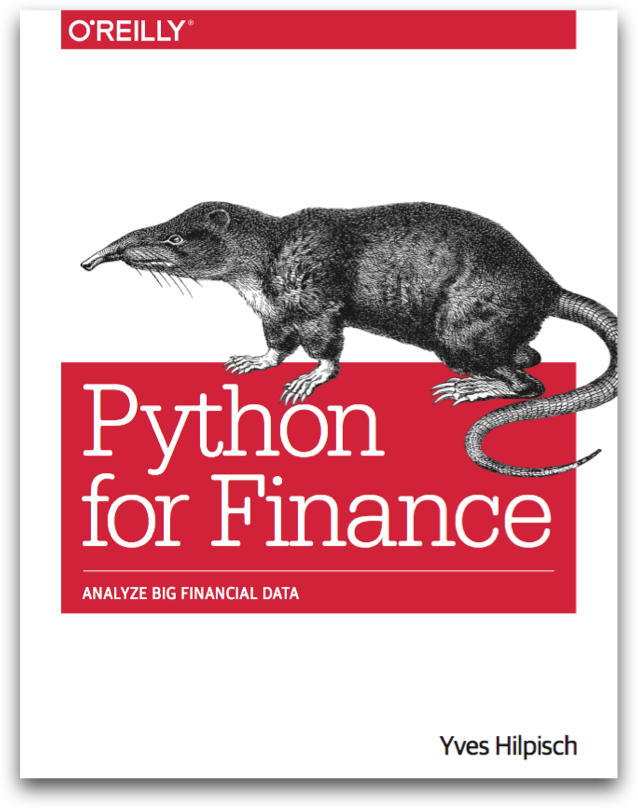Python for Finance (O'Reilly Shop)

## IPython¶

IPython Notebook is an interactive browser-based Python shell and "data exploration" environment. Among others it is characterized by the following features:

• interactive Python coding
• magic commands
• system shell
• markdown capabilities
• Latex support
• export capabilities (HTML, slides, Latex/PDF)
• parallel computing with IPython.parallel

As of today, IPython is the most popular and most powerful (?!) development environment for interactive Python development. It comes in three technological flavours:

• IPython Shell
• IPython QTConsole
• IPython Notebook

The only thing that you need in addition is a good text editor (my choice Sublime Text, http://www.sublimetext.com).

And a consistent, easy to use and maintain Python distribution (my choice Anaconda, http://continuum.io/downloads).

## NumPy – The Numerical Workhorse

NumPy represents the basic building of the whole scientific stack in Python. The major class is the ndarray class which models a multi-dimensional data container for array-like data (vectors, matrices, cubes, tables).

In :
import numpy as np

In :
data = np.random.standard_normal((50, 20))

In :
data[:, 1]
# indexing, slicing

Out:
array([ 0.88231997,  0.03204471,  0.87193974, -2.30564232,  1.27901701,
1.63420212,  0.19792775,  0.33824558,  0.59893164, -0.09839025,
-0.23429775,  0.0917838 , -0.73425413,  0.69589783, -0.65724823,
-2.13538985, -0.27524443,  1.5857643 , -0.15759166, -0.19029719,
-0.13815876,  0.99445934, -0.87108043, -1.23117082,  0.56235449,
0.66447094,  0.39993637, -1.36229192, -0.34697377, -0.08576895,
-0.04199554,  0.95540384,  0.31453524, -1.00648148,  0.0533431 ,
-0.45862602,  1.4772261 ,  0.82005407,  0.16435861,  0.6492746 ,
-0.60036387, -1.4964793 ,  0.61955265,  2.3517128 , -0.08469854,
-0.83197856,  0.26502647, -1.22434438, -0.84266327,  0.52352964])


NumPy provides powerful methods and so-called universal functions (all implemented in C for performance).

In :
data.mean()
# powerful methods ...

Out:
0.011431790549808587

In :
np.std(data)
# ... and universal functions (ufuncs)

Out:
1.03910742968812


All other major libraries, like matplotlib interact seamlessly with NumPy arrays.

In :
plt.plot(data.cumsum(axis=0))
plt.grid()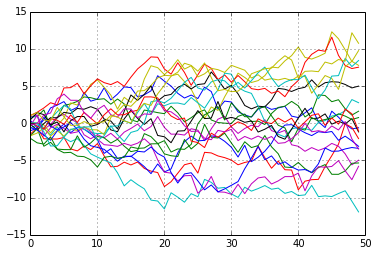## pandas – For Time Series Data and More

One major strength of the pandas library is the management and analysis of time series data.

In :
import pandas as pd

In :
index = pd.date_range(start='2014/7/1', periods=len(data), freq='W')
# define DatetimeIndex object

In :
index

Out:
<class 'pandas.tseries.index.DatetimeIndex'>
[2014-07-06, ..., 2015-06-14]
Length: 50, Freq: W-SUN, Timezone: None

In :
df = pd.DataFrame(data, index=index)
# define DataFrame object


pandas brings lots of convenience to data analysis.

In :
df.iloc[:, :3].describe()

Out:
0 1 2
count 50.000000 50.000000 50.000000
mean -0.012270 0.032238 -0.041415
std 1.052172 0.946058 1.185388
min -2.375038 -2.305642 -2.660056
25% -0.767524 -0.564929 -0.802402
50% 0.028035 0.042694 -0.033669
75% 0.616627 0.641844 0.659427
max 2.203526 2.351713 2.790909

8 rows × 3 columns

Typical ufuncs of NumPy can in general be applied to DataFrame objects. pandas also provides a convenience wrapper for the matplotlib library.

In :
df.cumsum().plot(legend=False)

Out:
<matplotlib.axes.AxesSubplot at 0x4417650>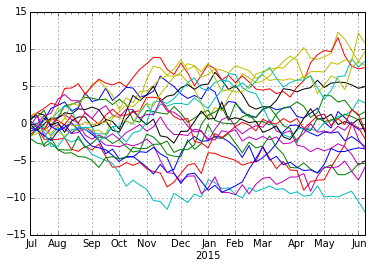## PyTables for Hardware Bound IO¶

PyTables is a Python wrapper for the high performing HDF5 file/database format (http://www.hdfgroup.org)

In :
import tables as tb


We work with a NumPy array containing 1 GB of random data.

In :
n = 12500
m = 10000

In :
%time data = np.random.standard_normal((n, m))

CPU times: user 8.93 s, sys: 163 ms, total: 9.09 s
Wall time: 9.1 s


In :
data.nbytes
# a giga byte worth of data

Out:
1000000000


PyTables reaches in general reading and writing speeds that are only bound by the performance of the hardware.

In :
h5 = tb.open_file(path + 'data.h5', 'w')

In :
%time h5.create_array('/', 'data', data)

CPU times: user 0 ns, sys: 1.17 s, total: 1.17 s
Wall time: 1.24 s


Out:
/data (Array(12500, 10000)) ''
atom := Float64Atom(shape=(), dflt=0.0)
maindim := 0
flavor := 'numpy'
byteorder := 'little'
chunkshape := None

In :
h5.get_filesize()

Out:
1000002304L

In :
h5.close()


Reading the data is generally pretty fast.

In :
h5 = tb.open_file(path + 'data.h5', 'r')

In :
%time data = h5.root.data.read()

CPU times: user 0 ns, sys: 387 ms, total: 387 ms
Wall time: 386 ms


In :
data.flags.owndata

Out:
True

In :
h5.close()


## Application – Optimal Mean-Variance Portfolios

Let us implement a Markowitz portfolio optimization procedure with the help of NumPy, pandas & PyTables.

In :
symbols = ['AAPL', 'GOOG', 'MSFT', 'DB', 'GLD']
# the symbols for the portfolio optimization
noa = len(symbols)


pandas provides functions to retrieve stock price information from eg Yahoo! or Google.

In :
import pandas.io.data as web

In :
data = pd.DataFrame()
for sym in symbols:
data.columns = symbols


pandas is also well integrated with PyTables for performant DataFrame storage and retrieval.

In :
h5 = pd.HDFStore(path + 'stocks.h5', 'w')

In :
%time h5['stocks'] = data

CPU times: user 11 ms, sys: 0 ns, total: 11 ms
Wall time: 10.1 ms


In :
h5.close()


A graphical inspection of the normalized stock price data.

In :
(data / data.ix * 100).plot(figsize=(8, 5))

Out:
<matplotlib.axes.AxesSubplot at 0x4b62a10>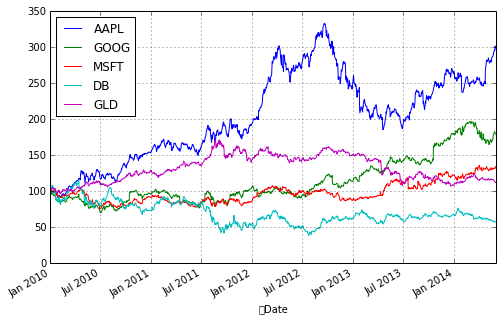For the portfolio analysis, return data is needed.

In :
rets = np.log(data / data.shift(1))

In :
rets.mean() * 252

Out:
AAPL    0.249753
GOOG    0.127497
MSFT    0.062951
DB     -0.127312
GLD     0.025235
dtype: float64


With pandas, the covariance matrix is only one method call away.

In :
rets.cov() * 252

Out:
AAPL GOOG MSFT DB GLD
AAPL 0.075210 0.028901 0.021544 0.043153 0.005840
GOOG 0.028901 0.064642 0.024298 0.048532 0.000941
MSFT 0.021544 0.024298 0.051330 0.048981 0.002308
DB 0.043153 0.048532 0.048981 0.185298 0.009495
GLD 0.005840 0.000941 0.002308 0.009495 0.033448

5 rows × 5 columns

The return of a portfolio is given as

$$\begin{eqnarray*} \mu_p &=& \mathbf{E}\left(\sum_I w_i r_i \right) \\ &=& \sum_I w_i \mathbf{E}\left( r_i \right) \\ &=& \sum_I w_i \mu_i \\ &=& w^T \mu \end{eqnarray*}$$

Here, $w_i$ and $r_i$ are the weight and the (expected, historical) return of asset $i$, respectively.

The covariance matrix is defined as follows.

$$\begin{eqnarray*} \Sigma = \begin{bmatrix} \sigma_{1}^2 \ \sigma_{12} \ \dots \ \sigma_{1I} \\ \sigma_{21} \ \sigma_{2}^2 \ \dots \ \sigma_{2I} \\ \vdots \ \vdots \ \ddots \ \vdots \\ \sigma_{I1} \ \sigma_{I2} \ \dots \ \sigma_{I}^2 \end{bmatrix} \end{eqnarray*}$$

Here, it holds $\sigma_{ij} = \sigma_{ji} = E\left(\left(r_i-\mu_i\right)\left(r_j-\mu_j\right)\right)$.

Given the covariance matrix, the portfolio variance is then given below.

$$\begin{eqnarray*} \sigma_p^2 &=& \mathbf{E}\left( (r - \mu )^2 \right) \\ &=& \sum_{i \in I}\sum_{j \in I} w_i w_j \sigma_{ij} \\ &=& w^T \Sigma w \end{eqnarray*}$$

The Python implementations are even a bit more compact than the mathematical definitions ...

In :
weights = np.random.random(noa)
weights /= np.sum(weights)
# getting random weights and normalization
weights

Out:
array([ 0.29176863,  0.32311079,  0.16928072,  0.01197019,  0.20386966])

In :
np.sum(weights * rets.mean() ) * 252
# expected portfolio return

Out:
0.12834274272498228

In :
np.dot(weights.T, np.dot(rets.cov() * 252, weights))
# expected portfolio variance

Out:
0.028173947646012686

In :
np.sqrt(np.dot(weights.T, np.dot(rets.cov() * 252, weights)))
# expected portfolio standard deviation/volatility

Out:
0.16785096855845869


We can easily simulate possible mean-variance/volatility combinations.

In :
%%time
prets = []
pvols = []
for p in range (2500):
weights = np.random.random(noa)
weights /= np.sum(weights)
prets.append(np.sum(rets.mean() * weights) * 252)
pvols.append(np.sqrt(np.dot(weights.T,
np.dot(rets.cov() * 252, weights))))
prets = np.array(prets)
pvols = np.array(pvols)

CPU times: user 2.98 s, sys: 0 ns, total: 2.98 s
Wall time: 2.98 s



Let us inspect the results graphically.

In :
plt.figure(figsize=(8, 4))
plt.scatter(pvols, prets, c=prets / pvols, marker='o')
plt.grid(True)
plt.xlabel('expected volatility')
plt.ylabel('expected return')
plt.colorbar(label='Sharpe ratio')

Out:
<matplotlib.colorbar.Colorbar instance at 0x5010830>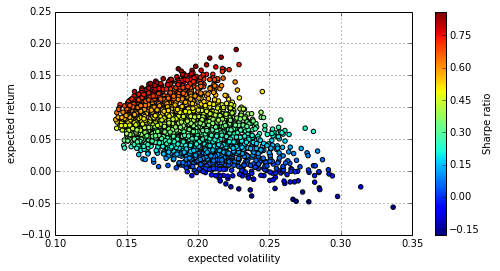Let us derive the efficient frontier. First, some helper functions.

In :
def statistics(weights):
pret = np.sum(weights * rets.mean()) * 252
pvol = np.sqrt(np.dot(weights.T, np.dot(rets.cov() * 252, weights)))
return np.array([pret, pvol])

In :
def min_portfolio_vola(weights):
return statistics(weights)


Second, the optimization procedure.

In :
import scipy.optimize as sco

In :
bnds = tuple((0, 1) for _ in xrange(noa))
# bounds for the asset weights

In :
%%time
trets = np.linspace(0.0, 0.21, 50)
tvols = []
for tret in trets:
cons = ({'type': 'eq', 'fun': lambda x:  statistics(x) - tret},
{'type': 'eq', 'fun': lambda x:  np.sum(x) - 1})
res = sco.minimize(min_portfolio_vola, noa * [1. / noa,], method='SLSQP',
bounds=bnds, constraints=cons)
tvols.append(res['fun'])
tvols = np.array(tvols)

CPU times: user 5.02 s, sys: 0 ns, total: 5.02 s
Wall time: 5.03 s



And the efficient frontier visualized.

In :
plt.figure(figsize=(8, 4))
plt.scatter(pvols, prets, c=prets / pvols, marker='o')
plt.scatter(tvols, trets, c=trets / tvols, marker='x')
plt.grid(True)
plt.xlabel('expected volatility')
plt.ylabel('expected return')
plt.colorbar(label='Sharpe ratio')

Out:
<matplotlib.colorbar.Colorbar instance at 0x5641ab8>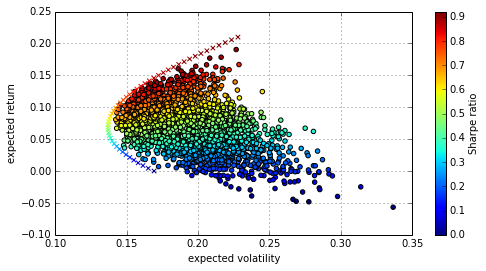## Application – Principal Component Analysis

Principal Component Analysis (PCA) is another important statistical tool in finance. Using the same libraries as before and a "bit more", we are going to implement a PCA based on the names in the German DAX index.

In :
from sklearn.decomposition import KernelPCA


First, we need the relevant stock price and index data.

In :
symbols = ['ADS.DE', 'ALV.DE', 'BAS.DE', 'BAYN.DE', 'BEI.DE',
'BMW.DE', 'CBK.DE', 'CON.DE', 'DAI.DE', 'DB1.DE',
'DBK.DE', 'DPW.DE', 'DTE.DE', 'EOAN.DE', 'FME.DE',
'FRE.DE', 'HEI.DE', 'HEN3.DE', 'IFX.DE', 'LHA.DE',
'LIN.DE', 'LXS.DE', 'MRK.DE', 'MUV2.DE', 'RWE.DE',
'SAP.DE', 'SDF.DE', 'SIE.DE', 'TKA.DE', 'VOW3.DE',
'^GDAXI']

In :
%%time
data = pd.DataFrame()
for sym in symbols:
data = np.log(data / data.shift(1))
# calculating the log returns
data = data.dropna()
# getting rid of rows with missing data

CPU times: user 289 ms, sys: 26 ms, total: 315 ms
Wall time: 3.37 s



We now have all the return data in a single DataFrame object.

In :
data[data.columns[:5]].head()

Out:
Date
2010-01-05 0.030937 0.003045 -0.015278 -0.018431 -0.005181
2010-01-06 -0.008089 0.007739 0.006319 -0.006341 -0.000650
2010-01-07 0.008592 -0.011575 -0.006772 -0.013173 -0.010232
2010-01-08 -0.003529 -0.005440 -0.002949 -0.008879 -0.029309
2010-01-11 0.006293 -0.011315 -0.011194 -0.000186 0.018529

5 rows × 5 columns

For convenience, we separate the DAX index data.

In :
dax = pd.DataFrame(data.pop('^GDAXI'))

In :
dax.hist(bins=35)

Out:
array([[<matplotlib.axes.AxesSubplot object at 0x602e990>]], dtype=object)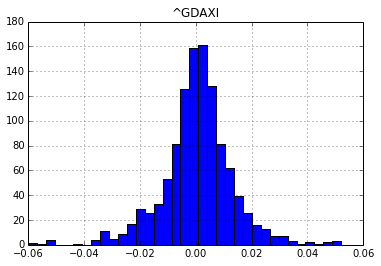Let us implement the PCA.

In :
scale_function = lambda x: (x - x.mean()) / x.std()

In :
pca = KernelPCA().fit(data.apply(scale_function))


Let's check the explanatory power of the first components.

In :
get_we = lambda x: x / x.sum()
# weighting function

In :
get_we(pca.lambdas_)[:10]
# explanatory power of first ten components

Out:
array([ 0.49037485,  0.0566472 ,  0.04729083,  0.03080232,  0.02800526,
0.02421227,  0.02369448,  0.02197784,  0.02039824,  0.02014176])

In :
get_we(pca.lambdas_)[:10].sum()
# power of first five components (>75%)

Out:
0.76354505762316349


A PCA index with 1 component replicating the DAX index.

In :
pca = KernelPCA(n_components=1).fit(data.apply(scale_function))
dax['PCA_1'] = pca.transform(-data)

In :
dax.cumsum().apply(scale_function).plot(figsize=(8, 4))

Out:
<matplotlib.axes.AxesSubplot at 0x575b5d0>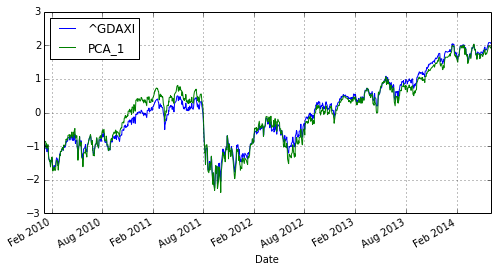A PCA index with 10 components replicating the DAX index.

In :
pca = KernelPCA(n_components=10).fit(data.apply(scale_function))
pca_components = pca.transform(data)
weights = get_we(pca.lambdas_)
dax['PCA_10'] = np.dot(pca_components, weights)

In :
dax[['^GDAXI', 'PCA_10']].cumsum().apply(scale_function).plot(figsize=(8, 4))

Out:
<matplotlib.axes.AxesSubplot at 0x61e3410>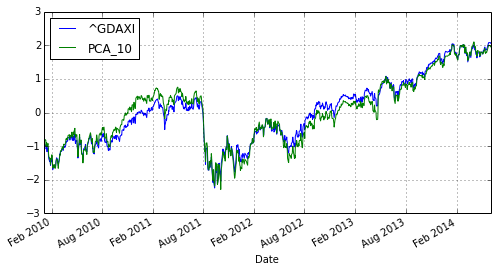## Parallel Process Simulation¶

Monte Carlo simulation is a computationally demanding task that nowadays is implemented generally on a large scale (eg for Value-at-Risk or Credit-Value-Adjustment calculations).

In :
import math


NumPy per se is well suited to implement efficient numerical algorithms, like Monte Carlo simulation.

In :
def simulate_geometric_brownian_motion(p):
M, I = p
# time steps, paths
S0 = 100; r = 0.05; sigma = 0.2; T = 1.0
# model parameters
dt = T / M
paths = np.zeros((M + 1, I))
paths = S0
for t in range(1, M + 1):
paths[t] = paths[t - 1] * np.exp((r - 0.5 * sigma ** 2) * dt +
sigma * math.sqrt(dt) * np.random.standard_normal(I))
return paths


Vectorization leads to compact code, C implementation under the hood to good performance.

A sample simulation with the simulation function.

In :
%time paths = simulate_geometric_brownian_motion((50, 100000))
# example simulation
paths[:, 0]

CPU times: user 573 ms, sys: 9 ms, total: 582 ms
Wall time: 582 ms


Out:
array([ 100.        ,  105.07666765,  100.05992252,   99.18123591,
94.56822829,   99.52323838,  100.76919114,  103.0287625 ,
102.89694065,  106.15871891,  108.38916813,  105.77019972,
104.91639211,  109.76683676,  110.51525631,  116.69665255,
116.41061171,  118.42839675,  119.90547802,  121.47917704,
117.72896608,  116.16695944,  118.20644679,  116.50127645,
116.29938684,  121.93312325,  126.07979317,  128.37206824,
127.72699949,  127.16388007,  123.40678122,  123.07624252,
124.17452649,  125.14144935,  125.97317885,  128.91712016,
135.84401375,  138.54173721,  139.50730126,  143.39303849,
137.98611154,  140.87751229,  143.33501052,  143.4872808 ,
140.58577668,  135.83654652,  134.66642245,  135.33467092,
132.52964714,  134.52802193,  138.05848016])

In :
paths[-1].mean()
# 5% drift p.a.

Out:
105.12017392735713


And a graphical look at selected data.

In :
plt.plot(paths[:, :10])
plt.grid(True)
# some paths visualized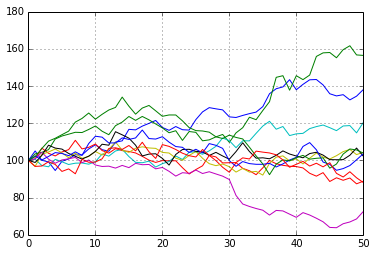In many financial application areas, not a single process but a larger number of processes has to be simulated. Such a task lends itself pretty well for parallelization.

In :
from time import time
import multiprocessing as mp

In :
I = 10000  # number of paths
M = 50  # number of time steps
t = 100 # number of tasks/simulations

In :
# running with 8 cores
times = []
for w in range(1, 9):
t0 = time()
pool = mp.Pool(processes=w)
result = pool.map(simulate_geometric_brownian_motion, t * [(M, I), ])
times.append(time() - t0)


A visual comparison of the results.

In :
plt.plot(range(1, 9), times)
plt.plot(range(1, 9), times, 'ro')
plt.grid(True)
plt.ylabel('time in seconds')
plt.title('%d Monte Carlo simulations' % t)

Out:
<matplotlib.text.Text at 0x6bfe250>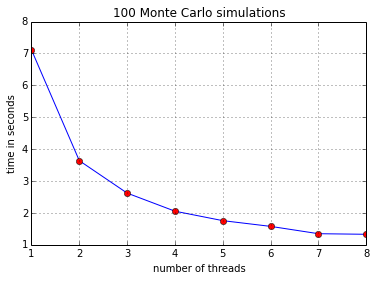## Parallel Monte Carlo Valuation¶

A major application area for Monte Simulation is option pricing. The multiprocessing approach translates pretty well to this application area.

In :
# European option class for simulation and valuation
class european_option(object):
import math
import numpy as np
def __init__(self, S0, T, r, sigma, h):
self.S0 = S0
self.T = T
self.r = r
self.sigma = sigma
self.h = h
def generate_paths(self, p):
M, I = p
dt = self.T / M
paths = np.zeros((M + 1, I))
paths = self.S0
for t in range(1, M + 1):
paths[t] = (paths[t - 1] *
np.exp((self.r - 0.5 * self.sigma ** 2) * dt +
self.sigma * math.sqrt(dt)
* np.random.standard_normal(I)))
return paths
def value_option(self, p, K):
paths = self.generate_paths(p)
payoff = eval(self.h)
return math.exp(-self.r * self.T) * np.sum(payoff) / p


Let us define a standard European call option.

In :
h = 'np.maximum(paths[-1] - K, 0)'
# payoff for standard European call option

In :
o = european_option(100, 1.0, 0.05, 0.2, h)
# the option object

In :
p = 50, 10000
# number of time steps, number of paths


The class allows, as before, the simulation of the geometric Brownian motion as well as the valuation of European options with arbitrary payoff.

In :
o.generate_paths(p)[:, 0]
# sample simulation

Out:
array([ 100.        ,   99.52372391,  101.14411958,  104.04738649,
100.5637259 ,   97.91671804,   97.17089487,  100.41933993,
101.30113598,  106.48142704,  102.75127199,  102.18797239,
105.50623455,  106.79967882,  108.5336573 ,  106.81750103,
108.49982205,  110.41185351,  110.15791632,  112.90730597,
113.85313159,  114.86243424,  117.23587079,  114.66527334,
112.6094626 ,  115.88649634,  128.34910768,  130.86995151,
129.35732195,  128.02680281,  135.85330458,  132.98754727,
138.52056831,  138.27533349,  141.05374387,  143.73180304,
143.98810523,  139.56188799,  143.25088076,  140.23302444,
139.01787839,  135.84301206,  134.41090737,  136.73615153,
139.87544883,  142.48957517,  144.23512344,  140.70636843,
134.1096993 ,  133.44734081,  129.59924446])

In :
o.value_option(p, 100)
# example valuation

Out:
10.720546637294582


Working with an instance of a class, we need to wrap methods in a function.

In :
# wrap method in function
def worker(K):
return o.value_option((50, 25000), K)

In :
times = []
for w in range(1, 9):
t0 = time()
pool = mp.Pool(processes=w)
result = pool.map(worker, range(80, 120))
# option values for strikes K from 80 to 120
times.append(time() - t0)


And the time results again compared graphically.

In :
plt.plot(range(1, 9), times)
plt.plot(range(1, 9), times, 'ro')
plt.grid(True)
plt.ylabel('time in seconds')
plt.title('%d Monte Carlo valuations' % len(result))

Out:
<matplotlib.text.Text at 0x6b08d90>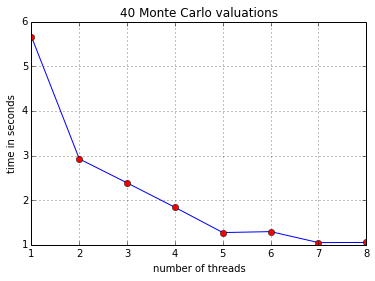## Processing Large CSV Files¶

We generate a CSV file on disk that is relatively large. We process this file with the help of pandas and PyTables.

First, some imports.

In :
import os
import numpy as np
import pandas as pd
import datetime as dt


### Generating an Example CSV File¶

Number of rows to be generated for random data set.

In :
N = int(1e7)
N

Out:
10000000


Using both random integers as well as floats.

In :
ran_int = np.random.randint(0, 10000, size=(2, N))
ran_flo = np.random.standard_normal((2, N))


Filename for csv file.

In :
csv_name = path + 'data.csv'


Writing the data row by row.

In :
%%time
try:
open(csv_name, 'r')
except:
with open(csv_name, 'wb') as csv_file:
for _ in xrange(10):
# 10 times the original data set
for i in xrange(N):
row = '%s, %i, %i, %f, %f\n' % \
(dt.datetime.now(), ran_int[0, i], ran_int[1, i],
ran_flo[0, i], ran_flo[1, i])
csv_file.write(row)
print os.path.getsize(csv_name)

597782528
1195565056
1793351680
2391134208
2988916736
3586703360
4184485888
4782272512
5380055040
5977837568
CPU times: user 19min 40s, sys: 4min 10s, total: 23min 51s
Wall time: 22min 41s



Delete the original NumPy ndarray objects.

In :
del ran_int
del ran_flo


Reading some rows to check the content.

In :
with open(csv_name, 'rb') as csv_file:
for _ in xrange(5):

date,int1,int2,flo1,flo2
2014-06-04 14:37:44.662453, 1691, 4136, -1.711282, 0.486395
2014-06-04 14:37:44.662489, 8971, 8196, -1.044905, 0.431430
2014-06-04 14:37:44.662504, 850, 8756, 1.622810, -1.102615
2014-06-04 14:37:44.662517, 7779, 5120, -0.770184, 1.034390



### Reading and Writing with pandas¶

The filename for the pandas HDFStore.

In :
pd_name = path + 'data.h5p'

In :
h5 = pd.HDFStore(pd_name, 'w')


pandas allows to read data from (large) files chunk-wise via a file-iterator.

In :
it = pd.read_csv(csv_name, iterator=True, chunksize=N / 20)


Reading and storing the data chunk-wise (this processes roughly 12 GB of data).

In :
%%time
for i, chunk in enumerate(it):
h5.append('data', chunk)
if i % 20 == 0:
print os.path.getsize(pd_name)

33118654
698528304
1363688670
2028738730
2693894904
3358922431
4024071885
4689231352
5354406128
6019346434
CPU times: user 3min 51s, sys: 15.2 s, total: 4min 6s
Wall time: 5min 14s



The resulting HDF5 file – with 100,000,000 rows and five columns.

In :
h5

Out:
<class 'pandas.io.pytables.HDFStore'>
File path: /flash/data/data.h5p
/data            frame_table  (typ->appendable,nrows->100000000,ncols->5,indexers->[index])


### Disk-Based Analytics with pandas¶

The disk-based pandas DataFrame mainly behaves like an in-memory object – but these operations are not memory efficient.

In :
%time h5['data'].describe()

CPU times: user 2min 39s, sys: 18.1 s, total: 2min 57s
Wall time: 2min 55s


Out:
int1 int2 flo1 flo2
count 100000000.000000 100000000.000000 100000000.000000 100000000.000000
mean 5000.182898 4999.851692 0.000314 -0.000653
std 2886.823750 2887.381294 0.999644 1.000313
min 0.000000 0.000000 -5.580576 -5.224685
25% 2499.000000 2499.000000 -0.673759 -0.674842
50% 5000.000000 5000.000000 -0.000136 -0.000887
75% 7501.000000 7501.000000 0.674500 0.673771
max 9999.000000 9999.000000 5.093366 5.280742

8 rows × 4 columns

Data selection and plotting works as with regular pandas DataFrame objects – again not really memory efficient.

In :
%%time
%matplotlib inline
h5['data']['flo2'][0:1000].cumsum().plot()

CPU times: user 31.4 s, sys: 6.32 s, total: 37.7 s
Wall time: 37.5 s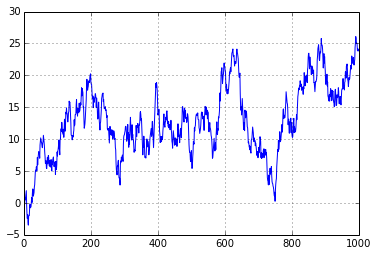In :
h5.close()


The major reason is that the DataFrame data structure is broken up (e.g. columns) during storage. For analytics it has to be put together in-memory again.

In :
import tables as tb
h5 = tb.open_file(path + 'data.h5p', 'r')
h5

Out:
File(filename=/flash/data/data.h5p, title='', mode='r', root_uep='/', filters=Filters(complevel=0, shuffle=False, fletcher32=False, least_significant_digit=None))
/ (RootGroup) ''
/data (Group) ''
/data/table (Table(100000000,)) ''
description := {
"index": Int64Col(shape=(), dflt=0, pos=0),
"values_block_0": Float64Col(shape=(2,), dflt=0.0, pos=1),
"values_block_1": Int64Col(shape=(2,), dflt=0, pos=2),
"values_block_2": StringCol(itemsize=26, shape=(1,), dflt='', pos=3)}
byteorder := 'little'
chunkshape := (1985,)
autoindex := True
colindexes := {
"index": Index(6, medium, shuffle, zlib(1)).is_csi=False}

In :
h5.close()


### Reading with pandas and Writing with PyTables¶

The PyTables database file.

In :
import tables as tb

In :
tb_name = path + 'data.h5t'

In :
h5 = tb.open_file(tb_name, 'w')


Using a rec array object of NumPy to provide the row description for the PyTables table. To this end, a custom dtype object is needed.

In :
dty = np.dtype([('date', 'S26'), ('int1', '<i8'), ('int2', '<i8'),
('flo1', '<f8'), ('flo2', '<f8')])
# change dtype for date from object to string


Adding compression to the mix (less storage, better backups, better data transfer, etc.).

In :
filters = tb.Filters(complevel=2, complib='blosc')


Again reading and writing chunk-wise, this time appending to a PyTables table object (this processes again roughly 12GB of uncompressed data).

In :
it = pd.read_csv(csv_name, iterator=True, chunksize=N / 20)

In :
%%time
tab = h5.create_table('/', 'data',
filters=filters)
# initialize table object by using first chunk and adjusted dtype
for chunk in it:
tab.append(chunk.to_records(index=False))
tab.flush()

CPU times: user 4min 24s, sys: 15.3 s, total: 4min 39s
Wall time: 4min 28s



The resulting table object – 100,000,000 rows and five columns.

In :
h5.get_filesize()

Out:
5800432765L

In :
tab

Out:
/data (Table(100000000,), shuffle, blosc(2)) ''
description := {
"date": StringCol(itemsize=26, shape=(), dflt='', pos=0),
"int1": Int64Col(shape=(), dflt=0, pos=1),
"int2": Int64Col(shape=(), dflt=0, pos=2),
"flo1": Float64Col(shape=(), dflt=0.0, pos=3),
"flo2": Float64Col(shape=(), dflt=0.0, pos=4)}
byteorder := 'little'
chunkshape := (9039,)


### Out-of-Memory Analytics with PyTables¶

Data on disk can be used as if it would be both in-memory and uncompressed. De-compression is done at run-time.

In :
tab[N:N + 3]
# slicing row-wise

Out:
array([ ('2014-06-04 14:40:00.801618', 1691, 4136, -1.711282, 0.48639499999999997),
('2014-06-04 14:40:00.801638', 8971, 8196, -1.044905, 0.43143000000000004),
('2014-06-04 14:40:00.801651', 850, 8756, 1.62281, -1.102615)],
dtype=[('date', 'S26'), ('int1', '<i8'), ('int2', '<i8'), ('flo1', '<f8'), ('flo2', '<f8')])

In :
tab[N:N + 3]['date']
# access selected data points

Out:
array(['2014-06-04 14:40:00.801618', '2014-06-04 14:40:00.801638',
'2014-06-04 14:40:00.801651'],
dtype='|S26')


Counting of rows is easily accomplished (although here not really needed).

In :
%time len(tab[:]['flo1'])
# length of column (object)

CPU times: user 599 ms, sys: 2.48 s, total: 3.08 s
Wall time: 3.02 s


Out:
100000000


Aggregation operations, like summing up or calculating the mean value, are another application area.

In :
%time tab[:]['flo1'].sum()
# sum over column

CPU times: user 1.37 s, sys: 2.39 s, total: 3.77 s
Wall time: 3.7 s


Out:
31414.387450003142

In :
%time tab[:]['flo1'].mean()
# mean over column

CPU times: user 1.38 s, sys: 2.4 s, total: 3.78 s
Wall time: 3.71 s


Out:
0.00031414387450003143


Typical, SQL-like, conditions and queries can be added.

In :
%time sum([row['flo2'] for row in tab.where('(flo1 > 3) & (int2 < 1000)')])
# sum combined with condition

CPU times: user 5.43 s, sys: 2.07 s, total: 7.51 s
Wall time: 5.23 s


Out:
321.51660000000021

In :
h5.close()


All operations have been on quite large data sets that might not fit (if uncompressed) into the memory of a single machine.

In :
ll /flash/data/*

-rw-r--r-- 1 root 5977841492  4. Jun 15:00 /flash/data/data.csv
-rw-r--r-- 1 root 1000002304  4. Jun 14:36 /flash/data/data.h5
-rw-r--r-- 1 root 6651285042  4. Jun 15:05 /flash/data/data.h5p
-rw-r--r-- 1 root 5800432765  4. Jun 15:13 /flash/data/data.h5t
-rw-r--r-- 1 root         33  4. Jun 14:36 /flash/data/save.pkl
-rw-r--r-- 1 root      61032  4. Jun 14:36 /flash/data/stocks.h5



Using compression of course reduces the size of the PyTables table object relative to the csv and the pandas HDFStore files.

In :
!rm /flash/data/*.h5*


## Conclusions¶

Python and libraries such as NumPy, pandas, PyTables provide useful means and approaches to work with (large, big) financial data. Using these libraries leads to a number of advantages:

• high level abstraction: Python provides "easy" access to advanced technologies; even non-expert programmers can become productive quite fast; the power of multi-core architectures is easily harnessed; similarly, analyst and developer productivity is relatively high in general
• numerical performance: libraries such as NumPy, pandas circumvent the performance burden of an interpreted language like Python by delegating performance-critical operations to routines implemented in C
• I/O performance: using PyTables which in turn relies on the HDF5 data storage standard allows to do IO at a speed only limited by the performance of the available hardware (eg 2/1 GB/s reading/writing speed with new SSDs from Intel)The Python Quants GmbH – the company Web site

www.pythonquants.com

Dr. Yves J. Hilpisch – my personal Web site

www.hilpisch.com

Python for Finance – O'Reilly (out as Early Release)

Python for Finance (O'Reilly)

Derivatives Analytics with Python – forthcoming at Wiley Finance

www.derivatives-analytics-with-python.com